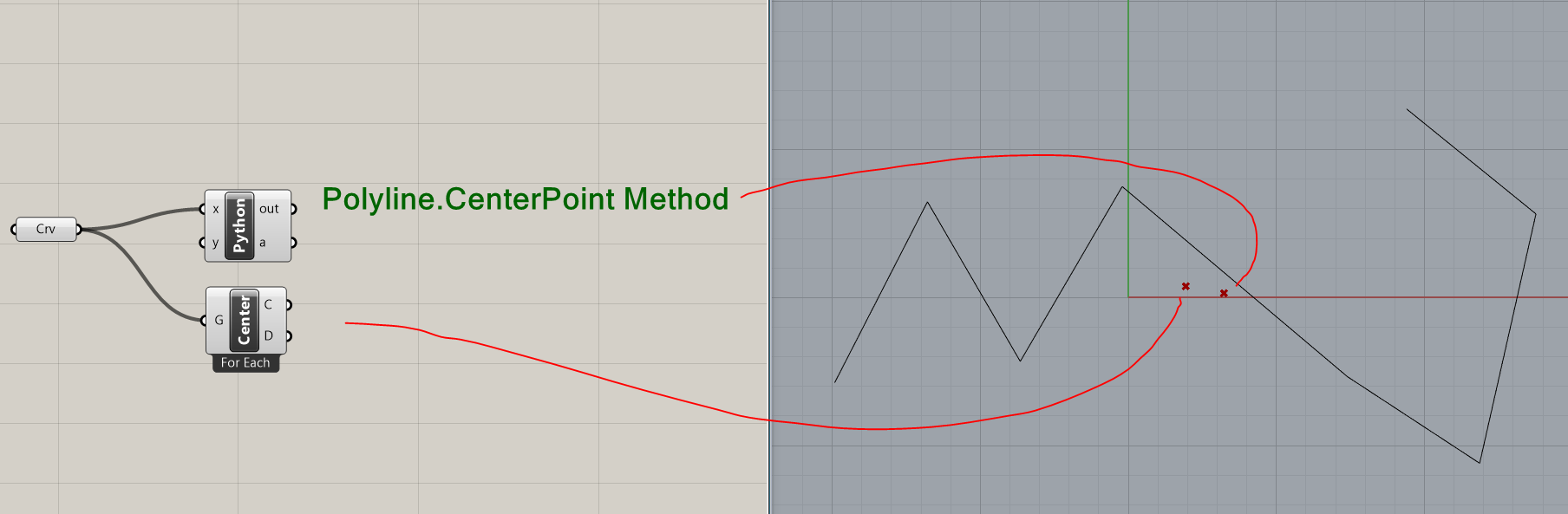# Polyline.CenterPoint() Math behind?

Hi,

Would it be possible to know what is the math behind the Polyline.CenterPoint() method
https://developer.rhino3d.com/5/api/RhinoCommonWin/html/M_Rhino_Geometry_Polyline_CenterPoint.htm

First I was thinking that it is simple addition of all points divided by number of points.
Point3d center = SumOfallPoints/numberOfAllPoints;

But it is not.

Length/2 maybe?Oh sorry it says weighted average of all segments

That is the question I have what does it mean by “weighted average of all segments” ?Yes I’ve noticed the difference too…not sure tbh

The logic behind is this:

1. get center point of each line segment multiplied by segment length
2. Sum up all those weighted points
3. Sum up all segment length

Somehow this center is much more nicer that typical average:

``````public Point3d CenterPoint(Polyline polyline)

{

int Count = polyline.Count;

if (Count == 0) { return Point3d.Unset; }

if (Count == 1) { return polyline; }

Point3d center = Point3d.Origin;

double weight = 0.0;

for (int i = 0; i < (Count - 1); i++)

{

Point3d A = polyline[i];

Point3d B = polyline[i + 1];

double d = A.DistanceTo(B);

center += d * 0.5 * (A + B);

weight += d;

}

center /= weight;

return center;

}``````

Yes weighted average. You can do the same with the weighted average component.